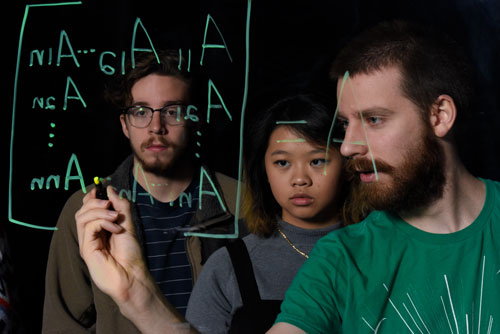# Applied Mathematics and Scientific Computation

This track is focused on providing our students with the mathematical and computational skills needed to develop and maintain mathematical models.

## What Is a Mathematical Model?A mathematical model is a rendering of some real-world system into the language of mathematics, usually taking the form of a single partial differential equation, or a system of such equations. The development of effective mathematical models is a fundamental need of our society, based as it is upon science and technology, and these models act as the indispensable link between us humans and the multitude of machines that we use to manage and investigate our world.

## How are Models Used?

In weather prediction, data is gathered globally and locally and used as input to a system of partial differential equations that include not only effects related to the atmosphere, but also oceanic and even polar effects. This system of equations is large and complex, and good mathematics together with powerful supercomputer systems are needed for their solution. The end result is that today hurricanes and even tornadoes, while not exactly predicable, are much more predicable than in earlier times. The early warning systems generated by these models have saved many lives in recent years.

There are many other examples of beneficial mathematical models. Many regions of the country depend crucially on groundwater for human and industrial/agricultural use. It is a renewable but fragile resource, and contamination and overuse is a constant problem. Once again, aquifers are modeled by a system of partial differential equations. The design of jumbo-sized jet aircraft, CAT-scan imaging in medicine, the effects of earth tremors on buildings in earthquake zones, and option pricing models in the stock market are some additional examples of mathematical models of value to society.

## Track Requirements

The Applied Mathematics and Scientific Computation Track includes a calculus and differential mathematics core. You will take courses like Scientific Programming, Introduction to Mathematical Modeling, and Modeling with Partial Differential Equations. Full requirements, a four-year plan, and course descriptions are available in the UAB Undergraduate Catalog.